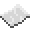# math.cosh

math.cosh
Function
Syntax
math.cosh(
• num : number
)

Returns number
API math
Source Lua (source)

Return the hyperbolic cosine of num.ExamplePrint the hyperbolic cosine of 1
Print the hyperbolic cosine of 1.
Code
```<nowiki>
print(math.cosh(1))
</nowiki>
```
Output 1.5430806348152